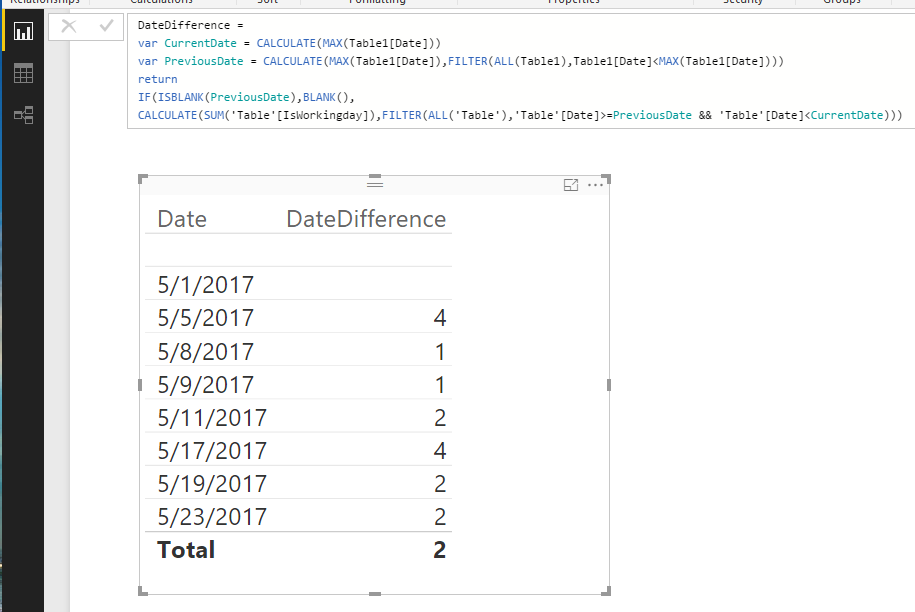cancel
Showing results for
Did you mean:

Fabric is Generally Available. Browse Fabric Presentations. Work towards your Fabric certification with the Cloud Skills Challenge.Helper I

## Find the difference between dates excluding Weekends

Hi,

I have to calculate the difference between two dates but excluding the weekends (Holiday's may in in future) i.e, Sat & Sun  for now.

I checked many threads here all the solutions are with seperate Date table and I don't have any seperate date table 😞1 ACCEPTED SOLUTIONMicrosoft

I have tested it on my local environment, we can add a calculated column to check if the date is working, and then sum up this calculated column.

Weekday = WEEKDAY('Table'[Date])
IsWorkingday = IF('Table'[Weekday]>6||'Table'[Weekday]<2,0,1)

DateDifference =
var CurrentDate = CALCULATE(MAX(Table1[Date]))
var PreviousDate = CALCULATE(MAX(Table1[Date]),FILTER(ALL(Table1),Table1[Date]<MAX(Table1[Date])))
return
IF(ISBLANK(PreviousDate),BLANK(),
CALCULATE(SUM('Table'[IsWorkingday]),FILTER(ALL('Table'),'Table'[Date]>=PreviousDate && 'Table'[Date]<CurrentDate)))Regards,

Charlie Liao

6 REPLIES 6Super User

Not much to go on here, but could you create a calculated column and use WEEKDAY() to get the day of the week. Let's say Sunday is 1 and Saturday is 7.

Then you could create a measure like the following:

`Measure = ([First Date] - [Second Date])*1. - CALCULATE(COUNT([Column]),FILTER(Table,([WeekDay] = 1 || [WeekDay = 7) && [Date] > [First Date] && [Date] < [Second Date]))`

Something along those lines.

Become an expert!: Enterprise DNA
External Tools: MSHGQM
Latest book!:
Mastering Power BI 2nd EditionDAX is easy, CALCULATE makes DAX hard...Helper I

@Greg_Deckler Thank you for your response.

For making it more simple I created a date table, and also created DayInweek as Saturday as 6 & Sunday as 7 and wrote something like you suggested:

I am creating a calculated column here.

`Total Time = 1.0*(Operations_Data[LAST DELIVERED EVENT] - Operations_Data[FIRST IN TRANSIT EVENT]) - CALCULATE(COUNT('Calendar'[IfWorkDay]),FILTER('Calendar','Calendar'[IfWorkDay] = 6 || 'Calendar'[IfWorkDay] = 7 ))`

But, I am not getting the proper result. Please suggestMicrosoft

I have tested it on my local environment, we can add a calculated column to check if the date is working, and then sum up this calculated column.

Weekday = WEEKDAY('Table'[Date])
IsWorkingday = IF('Table'[Weekday]>6||'Table'[Weekday]<2,0,1)

DateDifference =
var CurrentDate = CALCULATE(MAX(Table1[Date]))
var PreviousDate = CALCULATE(MAX(Table1[Date]),FILTER(ALL(Table1),Table1[Date]<MAX(Table1[Date])))
return
IF(ISBLANK(PreviousDate),BLANK(),
CALCULATE(SUM('Table'[IsWorkingday]),FILTER(ALL('Table'),'Table'[Date]>=PreviousDate && 'Table'[Date]<CurrentDate)))Regards,

Charlie LiaoHelper I

Thank you so much.

That works great. But I have one more question to ask may be you could answer:

I have 4 columns in my table:

First column

Second Column

Third Column

Fourth Column

I calculte the date diffeerence between First and Fourth but sometimes, First column has null values so insted of first I have to choose Second Column value and same with Third and Fourth (If Fourth is null, I have to choose Third Column value).

How could I proceed in the same example as you suggested above.Microsoft

You could use a IsBlank to check the column, such as

=IF(IsBlank(Table[Colum1]),Table[Column2],Table[Column1])

Regards,

Charlie LiaoSuper User

You could create two new columns, Five and Six with formulas like:

`Five = IF(ISBLANK([One]),[Two],[One])Six = IF(ISBLANK([Four]),[Three],[Four])`

Then just base everything off of columns Five and Six.

Become an expert!: Enterprise DNA
External Tools: MSHGQM
Latest book!:
Mastering Power BI 2nd EditionDAX is easy, CALCULATE makes DAX hard...Announcements#### Power BI Monthly Update - November 2023

Check out the November 2023 Power BI update to learn about new features.#### Fabric Community News unified experience

Read the latest Fabric Community announcements, including updates on Power BI, Synapse, Data Factory and Data Activator.#### Exclusive opportunity for Women!

Join us for a free, hands-on Microsoft workshop led by women trainers for women where you will learn how to build a Dashboard in a Day!#### The largest Power BI and Fabric virtual conference

130+ sessions, 130+ speakers, Product managers, MVPs, and experts. All about Power BI and Fabric. Attend online or watch the recordings.Top Solution Authors
Top Kudoed Authors
Users online (6,034)